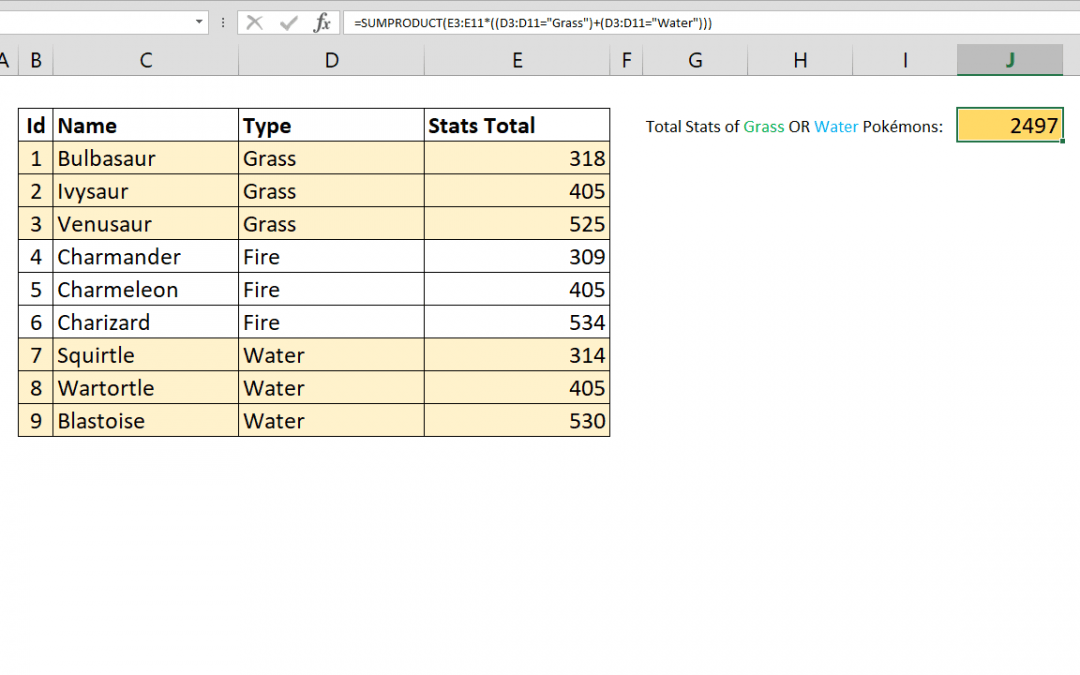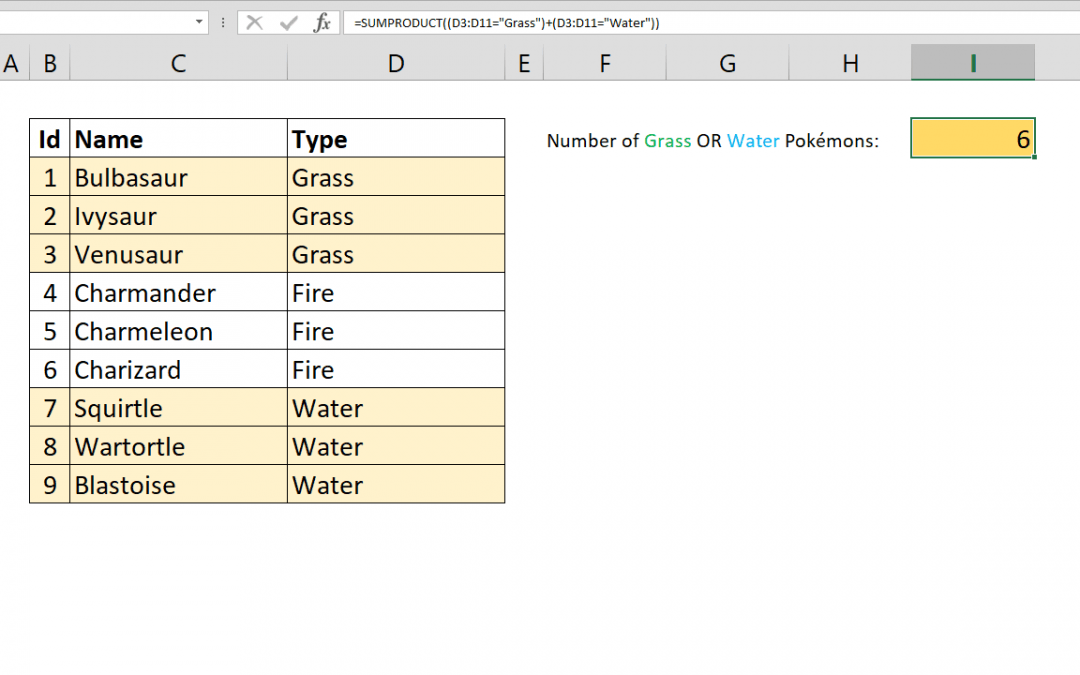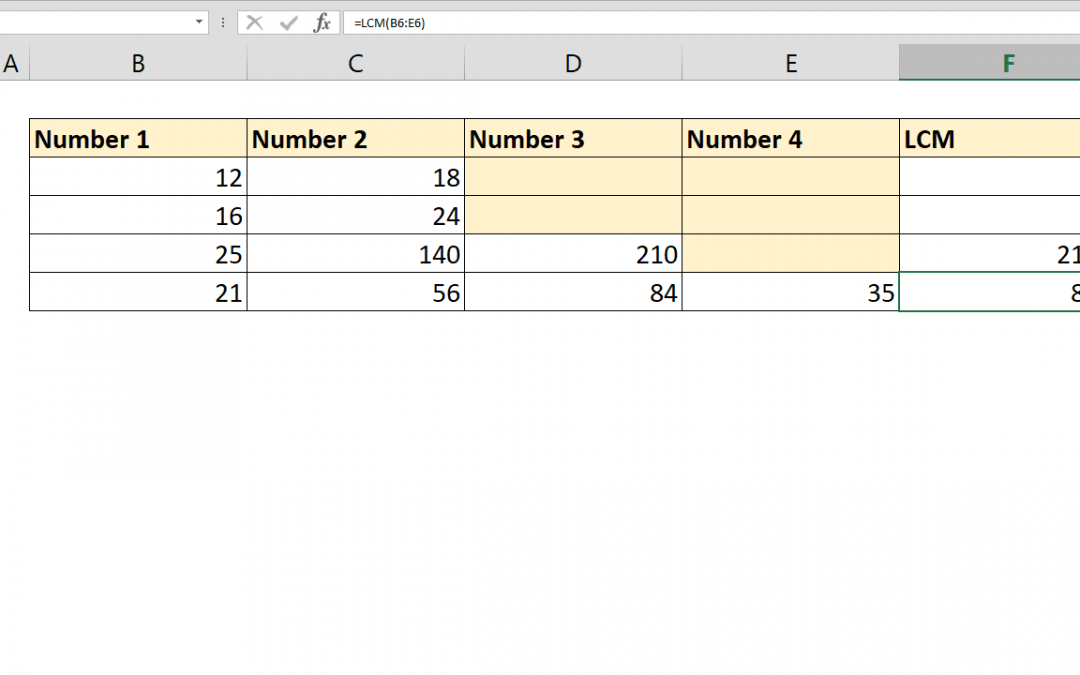## How to calculate average of values with OR operator

The AVERAGEIFS function can calculate the average of values with multiple conditions. However, these conditions are linked with AND operator. But if you want to handle AVERAGE OR combination, AVERAGEIFS function will not work. This article shows how to calculate...## How to sum values with OR operator using SUMPRODUCT with multiple criteria

The SUMIFS function can sum values based on multiple conditions. However, these conditions are all linked with AND operator. As a result, all conditions should be fulfilled to return a value. This article shows How you can sum values with multiple OR operators using...## How to count values with OR operator

You can count values in Excel by multiple criteria using the COUNTIFS function. However COUNTIFS will only link those criterion by AND operation. If you want to COUNTIFS OR, you need an alternative approach. This article shows how to count values with OR operator...## How to count characters in Excel in a range

The LEN function is used to count characters in Excel. But it doesn’t support ranges or arrays to easily count total number of characters in a range. This article shows 2 ways to count characters in Excel in a range which uses SUMPRODUCT and SUM along with LEN....## How to calculate Least Common Multiple

Excel has a built in function to calculate Least Common Multiple (LCM) of two or more integers. The function is also called LCM. Syntax =LCM(number1, [number2], …) Steps Start with =LCM( Type or select cells or range that includes integers B6:E6 Type ) to close the...## How to calculate Greatest Common Divisor (GCD)

To calculate Greatest Common Divisor (GCD) in Excel is very straightforward. Excel has a built in function to calculate the greatest common divisor of two or more integers. The function called GCD. Syntax =GCD(number1, [number2], …) Steps Start with =GCD( Type or...

## Windows Character Set (1252)

Windows Character Set is a one byte character encoding system derived from Latin alphabet. The character set shares same characters up to first 128 character with ASCII character encoding. Excel’s the CHAR function uses this characters set for Windows versions....

## MAC OS Character Set

Mac OS Character Set is based on one byte character encoding system, Mac-Roman. The character set shares same characters up to first 128 character with ASCII character encoding. Excel’s the CHAR function uses this characters set for Mac versions. Code...## How to use double quotes in Excel formulas

Excel uses double quote character to identify text, you need to a different approach. This article shows you How to use double quotes in Excel formulas in two ways: with and without a function. Syntax =”””” =CHAR(34) How There are 2 ways to use...